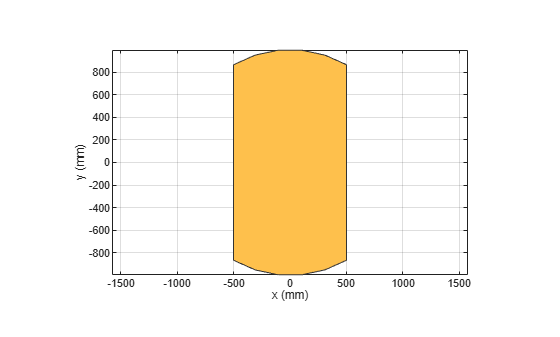# intersect

Boolean intersection operation on two shapes

## Syntax

``c = intersect(shape1,shape2)``

## Description

example

````c = intersect(shape1,shape2)` intersect `shape1` and `shape2` using the intersect operation. You can also use the `&` to intersect the two shapes.```

## Examples

collapse all

Create a default rectangle.

`r = antenna.Rectangle;`

Create a default circle.

`c = antenna.Circle;`

Use `intersect` to combine the shared surfaces of the rectangle and the circle.

`rc = intersect(r,c)`
```rc = Polygon with properties: Name: 'mypolygon' Vertices: [12x3 double] ```
```show(rc) axis equal```## Input Arguments

collapse all

Shapes created using custom elements and shape objects of Antenna Toolbox, specified as an object.

Example: `c = intersect(rectangle1, rectangle2)` where rectangle1 and rectangle2 are shapes created using `antenna.Rectangle` object.

## Version History

Introduced in R2017a